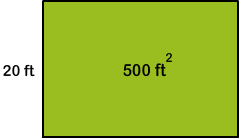# Area of Squares and Rectangles: Word Problems

12,589 Plays
Rate 0 stars
Common Core
Quiz size:
Message preview:
Someone you know has shared Area of Squares and Rectangles: Word Problems quiz with you:

To play this quiz, click on the link below:

https://www.turtlediary.com/quiz/area-of-squares-rectangles-word-problems.html

Hope you have a good experience with this site and recommend to your friends too.

Login to rate activities and track progress.
Login to rate activities and track progress.

The area is the amount of surface enclosed by a closed figure.

Area of a rectangle = length × width

Area of a square = side × side

For example, consider this situation.

The area of a rectangular pool is 500 square feet. The width of the pool is 20 feet.

Let's find the length of the pool.We know,

 Length × width = area Length × 20 = 500 Length = 500 ÷ 20 = 25

So, the length of the pool is 25 feet.

ds

A B C D E F G H I J K L M N O P Q R S T U V W X Y Z

### Help

##### Remember :

The smallest number is the one that comes first while counting.

##### Solution :

To arrange the given numbers in order from smallest to greatest, find the smallest number among all the given numbers.

21,27,23

21 is the smallest number.